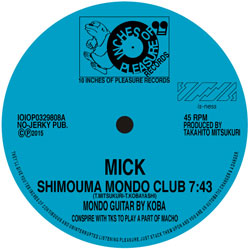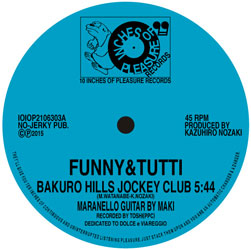# NLJNR20151111

#### NLJNR20151111

##### JAH-JAH-PLZ-JAH

10″のご案内になります。

###### 10 INCHES OF PLEASUREIOIOP0329808AIOIOP0329808B

[IOIOP0329808A/IOIOP2106303B] MICK – SHIMOUMA MONDO CLUB
[IOIOP2106303A/IOIOP0329808B] FUNNY&TUTTI – BAKURO HILLS JOCKEY CLUB

track listing
a1.Shimouma Mondo Club 7’43″

b1.Bakuro Hills Jockey Club 5’44″

###### 10 INCHES DOUBLE A-SIDER

10″ Split Single Spirit Double Sider

^v^v^v^v^v^v^v^v^v^v^v^v^v^v^v^v^v^v^v^v^v^v^v^v^v^v^v^v^v^v^v^v^v^v^v^v^v^v^v^v^v^v^v^v^v^v^v^v^v^v^v^v^v^v^v^v^v^v^v^v^v^v^v^v^v^v^v
One of the Setagaya Balearic style techno by Mick-Shimouma Mondo Club contained the mondo guitar by Takaomi Kobayashi as known as
ex.is-ness designer.
Also pleasure tribe new face Funny&Tutti-Bakuro Hills Jockey Club contained the maranello guitar by Maki(Liveloves)and this is one of the
disciple of G.Dolce’s Musica Meta-Meta Method before Midi era anni Ottanta.
^v^v^v^v^v^v^v^v^v^v^v^v^v^v^v^v^v^v^v^v^v^v^v^v^v^v^v^v^v^v^v^v^v^v^v^v^v^v^v^v^v^v^v^v^v^v^v^v^v^v^v^v^v^v^v^v^v^v^v^v^v^v^v^v^v^v^v

Presale:-JPY
Retail Price:Open